+ +

# MTF - Modulation Transfer Function

The optical performance of the human eye in terms of resolution and contrast sensitivity can be appreciated by a single function: the function of transfer of Modulation (FTM) or "Modulation transfer Function - MTF. Apart from the pure fundamental aspect that represents the understanding of the concepts of optics involved in its development, this setting opens clinical and therapeutic perspectives interesting and this article is therefore mainly dedicated to him.

### Introduction

The restoration of a good quality of vision is a major issue in ophthalmic surgery, and more particularly in the field of refractive surgery, and the cataract surgery. The measurement of Visual acuity at fort contrast (for example with black letters on background white, and which is quantified in ' number of tenths') is only one of the indicators of the quality of vision. This measure is very sensitive to the presence of a spherical defocus (myopia, hyperopia) or cylindrical (astigmatism); a demi-dioptrie of myopia is sufficient, for example, to reduce Visual acuity to maximum contrast of 2 decimal lines (loss of 2 tenths). On the other hand, certain aberrations of high degree (spherical aberration, coma) are compatible with the maintenance of Visual acuity under test in the conventional way, but induce a marked reduction in sensitivity to contrasts.

### Visual acuity with high contrast

The measurement of Visual acuity Foveal is one of the parameters to quantify the level of resolution of the Visual system. It corresponds to the inverse of the Visual angle necessary to distinguish the resolution (spatial frequency) the highest. This angle is called the 'angle of minimum resolution' (minimum angle of resolution: MAR). This angle, equivalent to the resolving power between two points just distinguished.

There are several kinds of Visual acuity (detection, separation, etc.). THEVisual acuity of separation explores the resolving power of the eye. This separator can be defined as the minimum angle for separating two different source points: angle MAR (Minimum Angle of Resolution). The calculation of the value of the MAR a theoretical look to take into account the phenomenon of pupillary diffraction. Visual acuity depends largely on the resolving power of the eye: to identify a letter projected white light on a dark screen, the eye can distinguish (separate) the grounds that form this letter. In these conditions, the contrast is maximum (in reality, close to 95%).

If the eye has no optical defect (or if that is perfectly corrected), resolution depends on diffraction, but also the size of the retinal photoreceptors: these sampling the retinal image. Their spatial density allows a theoretical acuity of around 20/10! In practice, this Visual acuity is rarely achieved because some optical defects are in addition to diffraction for the optical quality of the eye.

In comparison, the optical instruments used in astronomy (glasses, telescopes) have a resolving power that is significantly higher than that of the human eye. The resolving power of the Hubble telescope is about 1000 times higher than that of the human eye. Similarly, objectives which are digital cameras have an optical quality superior to that of the human eye: CMOS sensors that serve as their "retina" have a far superior definition - learn more about comparative performance of the eye and astronomical systems of bird-watching: Ophthalmology astronomy

### Conversion of the scales of acuity: tenths, cycles per degree, minutes of arc

#### Tenths and minutes of arc

In France, the opposite of Visual acuity in decimal notation is generally used: (A = 1/power splitter in minutes of arc).

A normal eye having a resolving power equal to one minute of arc has a Visual acuity of 1/1 = 1, or 10/10. Acuity of 0.1 (or 1/10) corresponds to a ten times less resolving power: the angle of resolution is equal to 10 minutes of arc.

For an eye with an acuity of 2.5/10, the angle measuring the resolving power of the eye is 1/4 or 4 minutes of arc (Visual acuity is divided by 4 compared to the threshold of reference of a minute of arc).

#### Tenths and cycles per degree

A cycle corresponds to the meeting a clear motive and a dark pattern. The intensity profile is sinusoidal.  The MAR angle is the angle in which you can register half a cycle (the clear part and the dark part of the cycle are just resolved).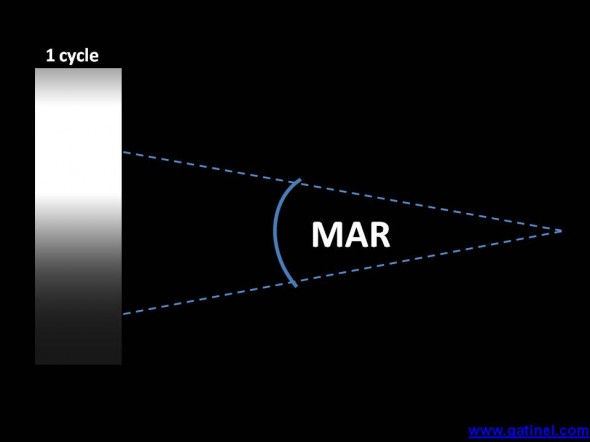MAR angle allows to solve a cycle if it registered its minimum and maximum of lumiance at an angle equal to the Mar. It takes two MAR to embrace the entire lifecycle

If the MAR is one minute, this corresponds to 1/60th of a degree, so we can register 30 resolved cycles in an angle of 1 degree (60 times the MAR so 60 times 1/2 cycles or 30 cycles).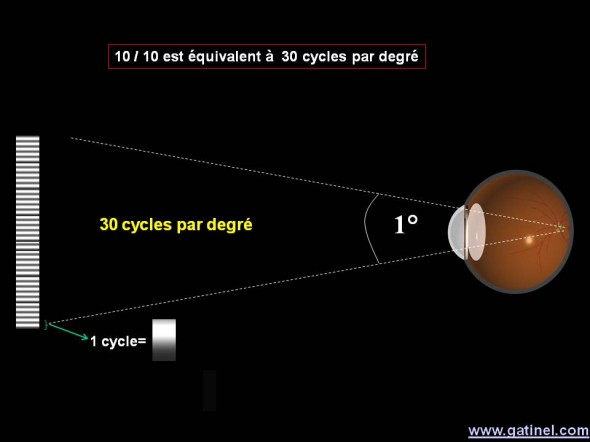A Visual acuity of 10/10 corresponds to the resolution of 30 cycles per degree (a cycle occupies in this case twice the space that embraces the value of the MAR angle, which is 1 minute of arc).

This unit allows to define the spatial frequencies, which are expressed in number of cycles per degree.

Values of 15/10, or even 20/10 (60 cycles per degree) are observed in practice in some subjects normalsighted or perfectly corrected. In this case, the eye "works" at the limit of capacity of sampling of the retina. The size of the retinal image of an 'E' is 15 microns surroundings: each of the "bars" of the E is projected onto a row of retinal cones. The E can be likened to 2 cycles and a half (spatial frequency: 60 cycles per degree, since the MAR is 30 seconds of arc and can enter 120 MAR a degree).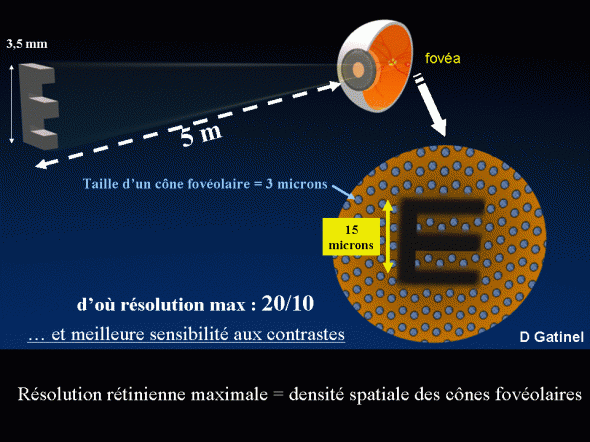The density of the mosaic of the retinal photoreceptors allows a maximum theoretical resolution of 20/10 or 30 seconds of arc (Tue) or 60 cycles per degree. In this example, the spacing between the centers of two photoreceptors is close to 2.5 microns

In clinical practice, the measure of Visual acuity is at maximum contrast; the existence of a variability of the lighting in the room where measures are performed or sharpness projectors explains that we can observe a change in the measure of Visual acuity for the same patient. You can intentionally seek this variation to measure Visual acuity in dynamic situation for example and establish the contrast sensitivity function.

The measurement of Visual acuity in high contrast (conducted e.g. by optotype black on white background) does not have a comprehensive indicator of the quality of vision. However, it is very sensitive to the presence of a spherical defocus (myopia, hyperopia) or cylindrical (astigmatism); a myopic defocus demi-dioptrie enough for instance to reduce Visual acuity to maximum contrast of 2 decimal lines. On the other hand, certain aberrations of high degree (spherical aberration, coma) are compatible with the maintenance of Visual acuity under test in the conventional way, but induce a marked reduction in sensitivity to contrasts.

The modulation of the contrast of the retinal image by the optical aberrations of the eye corresponds to the modulation transfer function (FTM); the acronym corresponding (MTF), which are more general, will be used here.

### Definition of the MTF

The MTF or FTM (function of transfer of Modulation) represents the way in which the optical system reduces the contrast of the image it forms compared to that of the object observed.

This indicator can be calculated from the study of aberrations distorting the wave front. It applies to any isolated optical system (cornea, implant) or a system consisting of different lenses (eye, lens of camera, telescope, telescope)...

To understand the interest of the MTF, it is necessary to know the properties of the decomposition of an image into basic components.

#### Image decomposition, convolution

The decomposition of an image into a set of basic point is quite intuitive (ex: pixels). Each point in the image observed is defined as a point of given intensity light source. If you know how the optical system treats the image of a point 'basic' (spread of the point or EFF function), then it is possible to apply this change to all the points composing the original image to get a simulation of the rendered image. This is called convolution of the image by the point spread function (Point Spread Function: PSF).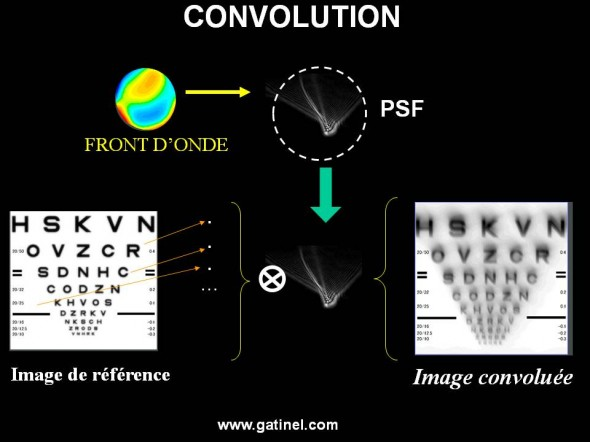The convolution is performed from the calculation of the PSF technique to predict the characteristics of the perceived image. Each point of the original image is assigned to the deformation of the PSF (convolution). The final image is obtained by recombination of convolues points.

#### Image and spatial frequencies

A monochrome image can also be decomposed into a combination of spatial frequencies: each of these frequencies is a network composed of strips alternately dark and light (monochromatic light), which may be oriented in a variable way within the image. A cycle is a pair of these bands (one dark, the other clear).

The number of pairs of bands darks and claires per unit of angle (cycles per degree) defined the value of the spatial frequency.

Each of the frequencies present in the decomposition of the image is weighted by a value that reflects its "amplitude", IE the difference in luminance between the darkest and brightest of the network.

The superposition of these different networks of spatial frequency to recompose the fixed image.

The fact that a picture can be broken down into a set of basic periodic signals called spatial frequency results from Fourier analysis that applies to almost any complex signal. However, this approach, familiar to the physicist, the electronics or the expert in signal processing is less ordinary for the clinician, more used to represent a Visual pattern as the juxtaposition of light points.

In order to make it more familiar, this method can be likened to the spectral decomposition of a sound in different sound frequencies. These spatial frequency spectrum extends from lower (the "serious" which correspond to the roughest elements of the image), the most high ('treble', allowing the representation of fine details). The MTF calculated for all of the eye diopters can be reconciled to the Visual function of the audiometric curve (audiogram) where it attaches to study hearing sensitivity to determine the threshold intensity of perception for each sound frequency.

Review current Visual acuity is exploring the ability of horizontal eye resolution for a maximum contrast target and is limited to information contained on the horizontal axis of the MTF. He would return for an audiometric examination only to test the hearing perception of different sound frequencies at maximum volume.

The spatial frequencies, in number of cycles/degree angle, are in category of the MTF curve. More cycles per degree, the more the spatial frequency is fine.

The spatial frequencies have a variable effect for visual perception. It showed, for example, that the recognition of patterns such as faces or the familiar landscapes is essentially from the right perception of medium spatial frequencies.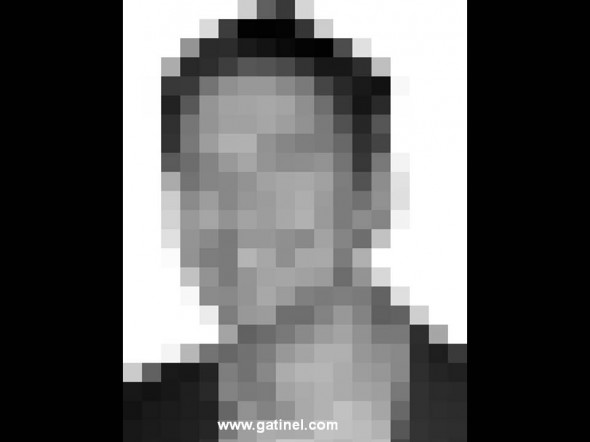The pixelated image represented here has high spatial frequencies, which correspond to the sharp edges of the square pixels. These frequencies interfere with the face recognition. Using a high frequency filter (observation image squinting, through an inappropriate correction glass, or long-distance) allows a better perception of the more average spatial frequencies and the possible recognition of the represented here effigy (which is none other than the author of this site). The perception of fine detail is not always beneficial for visual perception.

This notion echoes the relative tolerance of some older people towards a moderate myopic ametropia that does them little or no in their lives to identify relatives or find their way in a familiar universe. Moreover, the neuro-ophthalmologic treatment of Visual information acts as a filter, by reducing the perception of low spatial frequencies for the benefit of the mid frequencies.

### Interpretation of MTF curve

The MTF is the ratio between the respective contrasts of the image formed with the object attached to each spatial frequency: this value is carried in y, and corresponds to the modulation contrast (mitigation in percentage). Based on diffraction and the importance of the optical aberrations of the system being studied, it is possible to determine how the optical system reduces the contrast of any particular spatial frequency, and thus deduce the optical quality of the rendered image. More in this report is close to 100%, the better is the optical quality of the system tested for this spatial frequency.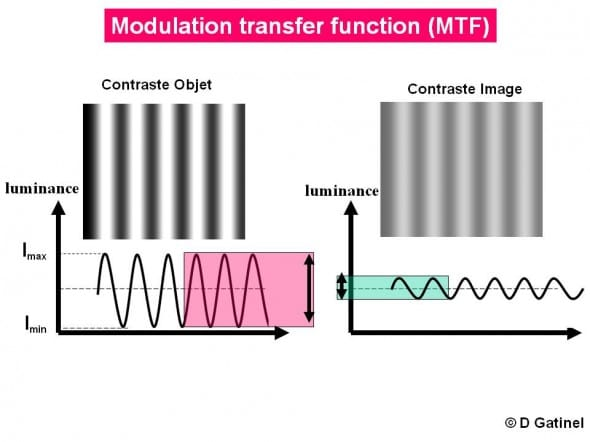A vertical network corresponding to a given spatial frequency sinusoidal is a fixed object. After refraction by the eye optical surfaces, the network to a contrast is reduced. The MTF represents the loss of contrast for each spatial frequency. The modulation is equal to: Modulation (M) = (Imax - Imin) / (Imax + Imin) the MTF is the relationship between Modulation of the image = / Modulation of the object. It is this value that gives the value of the ordinate of the MTF curve for a spatial frequency given.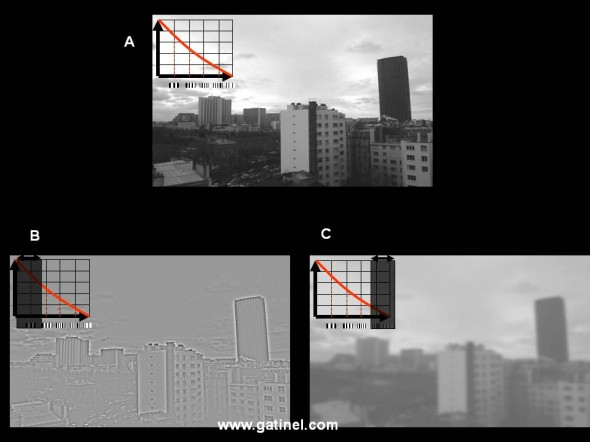Images and spatial frequencies. The reference (Tower and cemetery Montparnasse, Paris) image is made up of different low, medium, and high spatial frequencies (A). The 'rude' elements like the facades of the buildings at low and medium frequencies. Visible details within these facades are made by the high spatial frequencies. The transfer of these frequencies contrast varies: it is higher for low frequencies, and decreases towards the high frequencies (red curve). In B, the image was amputated the low spatial frequencies and a part of the mid frequencies. Most of the details are still noticeable, but the image lacks singularly 'contrast '. In C, the image is private space frequency high; some fine details have disappeared from the picture. A review of current Visual acuity (done at maximum contrast) of a patient seeing "and B" would not highlight of losses.

#### Cutoff frequency

A Visual acuity of 10/10th roughly corresponds to the resolving power to discern a spatial frequency of 30 cycles per degree. The intersection between the MTF curve and the axis of the abscissa corresponds to the cut-off frequency, IE the maximum theoretical acuity: the maximum theoretical frequency of the eye is 120 cycles per degree (a decimal Visual acuity of 20/10). case of myopia, the cut-off frequency decreases (ex: If myopia penalizes Visual acuity in high contrast to 2/10 max, theoretically in report frequency will be 6 cycles per degree; in reality, it may be less, because measuring Visual acuity is "psycho-Physics", whereas the calculation of the MTF is theoretical). In ophthalmology, the interest of the MTF is to study the effect of the only aberrations of high degree: the cut-off frequency can be near superior to 30 cycles per degree.

#### Aspect of the MTF curve

For an optical system 'perfect', devoid of optical aberrations, the MTF curve is a line of negative slope, because of the effects of diffraction which reduces the contrast of the high spatial frequencies. The presence of optical aberration of high degree reduces "the height" of the curve because these aberrations reduce the transmitted contrast. The surface below the MTF curve decreases.
Here is an example of MTF curve. Abscissa is the (lower left) spatial frequencies, higher on the right. The corresponding Visual acuity values are also reported. The letters E are appear to explain the fact that the MTF is a curve that allows to quantify clinical loss of contrast for different 'letter sizes.
Y is the value of the contrast modulation (it comes in this example of the lost contrast). There is an analogy between the sensitivity to the contrasts, and the MTF. the MTF explores that the contrast of the retinal image, which conditions, but it differs from the perceived image (cortical integration).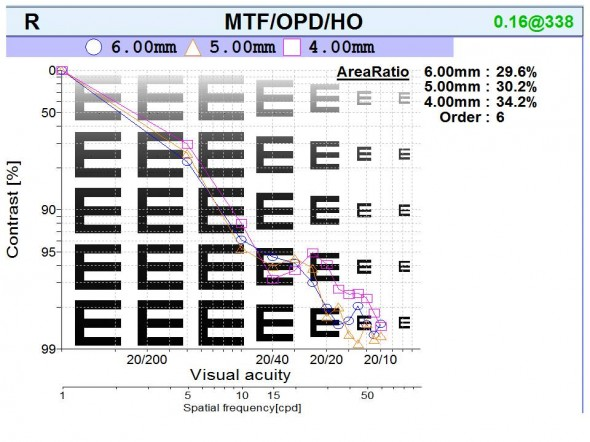MTF curves for different pupillary diameter (6mm, 5 mm and 4 mm) obtained after aberrometrique (OPDscan III, Nidek) measurement in a patient of 46, after cataract surgery. The area under each of curve is reported as a percentage: the percentage is relative to an 'average' MTF curve (established from measurements on a sample of normal eyes). Retinal modulation transfer reduction was related in this case a complicated surgery and a shift of the implant, originally of the optical aberrations of high degree rise. The rate and type of these aberrations however allows a near cutoff of 20/10, the highest being obtained for the lower diameter of pupil.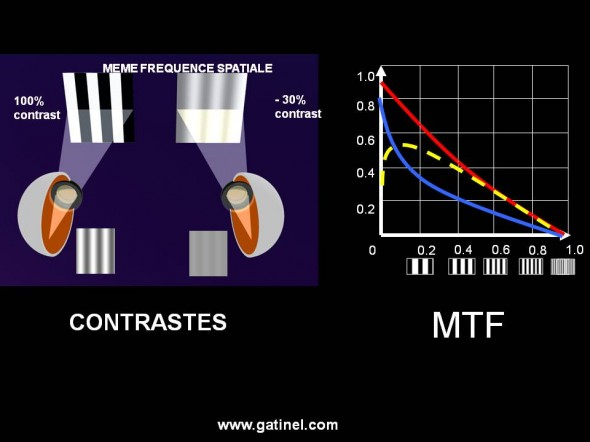A schematic representation of a MTF curve right.  the red line is a better visual envelope than the blue curve, while the resolution to maximum contrast is identical. The gap between the curves match the optical penalisation typically induced by an aberrations such as the spherical aberration. The MTF is based on the collection of the wave front. Vision of contrasts (schematic representation dotted yellow) corresponding to the type of patient no pathological history and presenting the MTF traced in red often reveals a lower sensitivity at low spatial frequencies than expected by the simple optical calculation. This reflects the integration of optical information by retinal receptors and Visual channels.